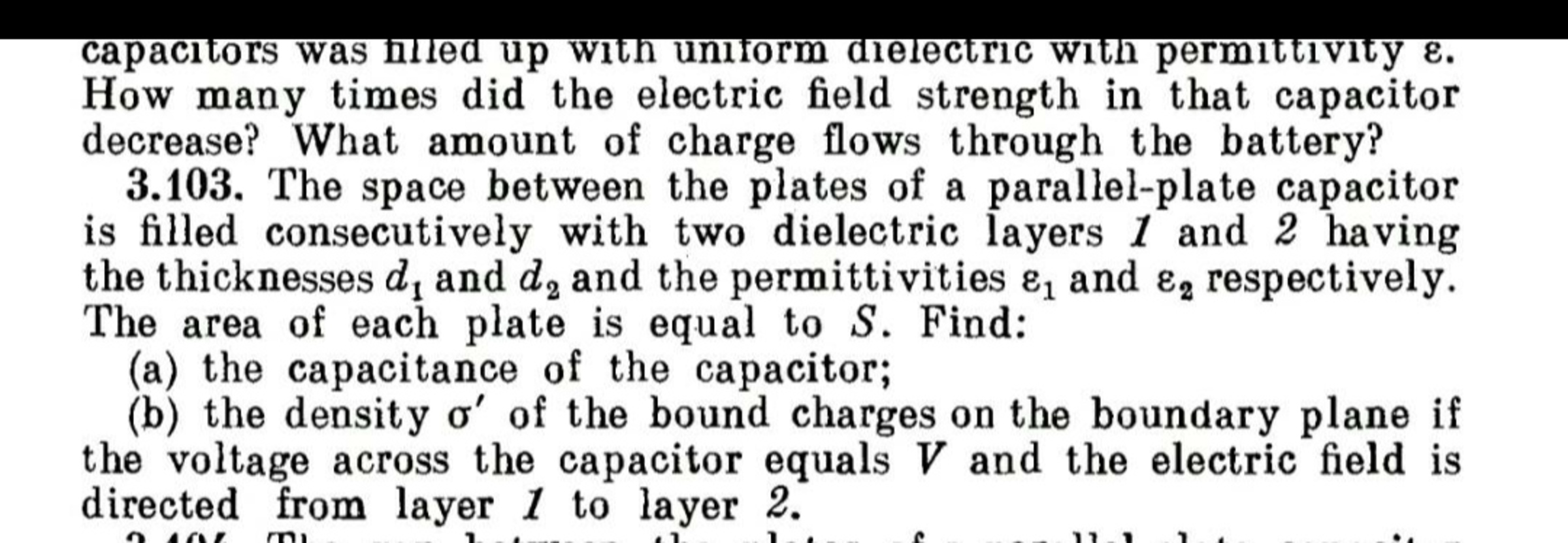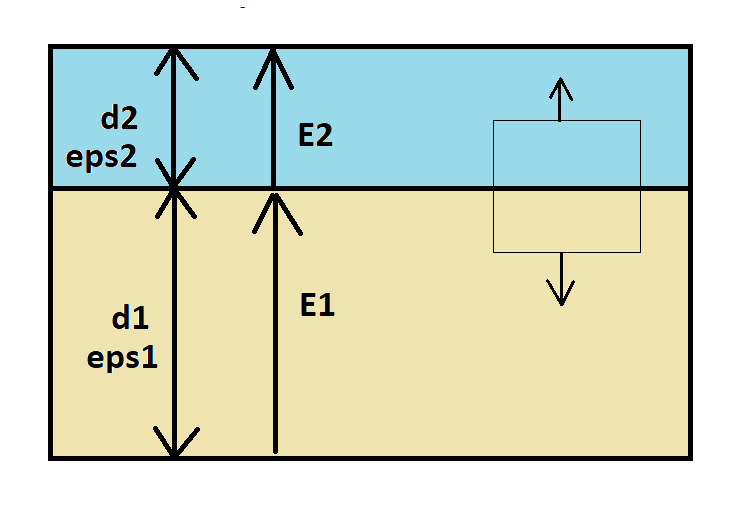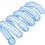# Bound Charge at Dielectric Interface

Here is a question sent to me recently:I will look at part (b). Let $\epsilon_1$ and $\epsilon_2$ be the relative electric permittivity values. The field continuity equation is (where the electric fields are perpendicular to the interface boundary):

$\epsilon_1 E_1 = \epsilon_2 E_2$

Relate the voltage across the capacitor to the electric fields:

$V = E_1 d_1 + E_2 d_2 = E_1 \Big(d_1 + \frac{\epsilon_1}{\epsilon_2} d_2 \Big) \\ E_1 = \frac{V \epsilon_2}{d_1 \epsilon_2 + d_2 \epsilon_1} \\ E_2 = \frac{V \epsilon_1}{d_1 \epsilon_2 + d_2 \epsilon_1}$

Consider the Gaussian surface shown to the right within the dielectric. The formula for bound charge at a dielectric interface is similar to the formula for Gauss's Law, but with a slight variation. Surfaces $S_2$ and $S_1$ are the top and bottom Gaussian sub-surfaces.

$Q_b = (\epsilon_1 - 1) \epsilon_0 \int \int_{S1} \vec{E}_1 \cdot \vec{dS_1} + (\epsilon_2 - 1) \epsilon_0 \int \int_{S2} \vec{E}_2 \cdot \vec{dS_2}$

Note that the normal vector for $S_1$ points in the opposite direction as the electric field, and the normal vector for $S_2$ points in the same direction as the electric field. Let these surfaces both have area $S$. The equation simplifies to:

$Q_b = (\epsilon_1 - 1) \epsilon_0 (- E_1 S) + (\epsilon_2 - 1) \epsilon_0 (E_2 S)$

Applying the field continuity relationship makes the relative permittivity terms disappear.

$Q_b = \epsilon_0 (E_1 S) - \epsilon_0 (E_2 S)$

Dividing both sides by $S$ gives the bound charge density:

$\sigma' = \epsilon_0 E_1 - \epsilon_0 E_2 = \epsilon_0 \Big( \frac{V \epsilon_2 - V \epsilon_1}{d_1 \epsilon_2 + d_2 \epsilon_1} \Big)$Note by Steven Chase
10 months, 1 week ago

This discussion board is a place to discuss our Daily Challenges and the math and science related to those challenges. Explanations are more than just a solution — they should explain the steps and thinking strategies that you used to obtain the solution. Comments should further the discussion of math and science.

When posting on Brilliant:

• Use the emojis to react to an explanation, whether you're congratulating a job well done , or just really confused .
• Ask specific questions about the challenge or the steps in somebody's explanation. Well-posed questions can add a lot to the discussion, but posting "I don't understand!" doesn't help anyone.
• Try to contribute something new to the discussion, whether it is an extension, generalization or other idea related to the challenge.
• Stay on topic — we're all here to learn more about math and science, not to hear about your favorite get-rich-quick scheme or current world events.

MarkdownAppears as
*italics* or _italics_ italics
**bold** or __bold__ bold
- bulleted- list
• bulleted
• list
1. numbered2. list
1. numbered
2. list
Note: you must add a full line of space before and after lists for them to show up correctly
paragraph 1paragraph 2

paragraph 1

paragraph 2

[example link](https://brilliant.org)example link
> This is a quote
This is a quote
    # I indented these lines
# 4 spaces, and now they show
# up as a code block.

print "hello world"
# I indented these lines
# 4 spaces, and now they show
# up as a code block.

print "hello world"
MathAppears as
Remember to wrap math in $$ ... $$ or $ ... $ to ensure proper formatting.
2 \times 3 $2 \times 3$
2^{34} $2^{34}$
a_{i-1} $a_{i-1}$
\frac{2}{3} $\frac{2}{3}$
\sqrt{2} $\sqrt{2}$
\sum_{i=1}^3 $\sum_{i=1}^3$
\sin \theta $\sin \theta$
\boxed{123} $\boxed{123}$

## Comments

Sort by:

Top Newest

@Steven Chase Hello, hope you are doing well
The formula which you have used for $Q_{b}$ is it a original formula for finding bound charges ?

- 7 months, 1 week ago

Log in to reply

@Steven Chase $Hel^{2}0$ I was again solving this question because I was doing revision.
And i just got stuck in your first line. I have forgot the concept little bit.
That line $\epsilon_{1}E_{1}=\epsilon_{2}E_{2}$
Just give me the proof of this line. Thanks in advance.
Hope I am not disturbing you.

- 8 months, 2 weeks ago

Log in to reply

In the absence of free charge, the permittivity-weighted normal components of the electric field are continuous at a dielectric interface. Here is a further reference:

https://en.wikipedia.org/wiki/Interfaceconditionsforelectromagneticfields

- 8 months, 1 week ago

Log in to reply

@Steven Chase Sir can you please post a RLC problem . I think due to some reason you are upset with me. If yes then sorry. If no, then why you don't reply me?

Log in to reply

There's a new one up now. I usually don't know when I'm going to post another one until just before I post it.

- 10 months ago

Log in to reply

@Steven Chase Thankyou sir

- 10 months, 1 week ago

Log in to reply

That's impressive. You found it ten seconds after I uploaded it

- 10 months, 1 week ago

Log in to reply

×

Problem Loading...

Note Loading...

Set Loading...